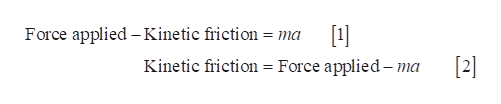# A horizontal force of 92.7 N is applied to a 54.5 kg crate on a rough, level surface. If the crate accelerates at 1.23 m/s2, what is the magnitude of the force of kinetic friction (in N) acting on the crate?

Question
9 views

A horizontal force of 92.7 N is applied to a 54.5 kg crate on a rough, level surface. If the crate accelerates at 1.23 m/s2, what is the magnitude of the force of kinetic friction (in N) acting on the crate?

check_circle

Step 1

The equation of motion along the...help_outlineImage TranscriptioncloseForce applied - Kinetic friction 1] = ma  Kinetic friction = Force applied-ma fullscreen

### Want to see the full answer?

See Solution

#### Want to see this answer and more?

Solutions are written by subject experts who are available 24/7. Questions are typically answered within 1 hour.*

See Solution
*Response times may vary by subject and question.
Tagged in

### Friction